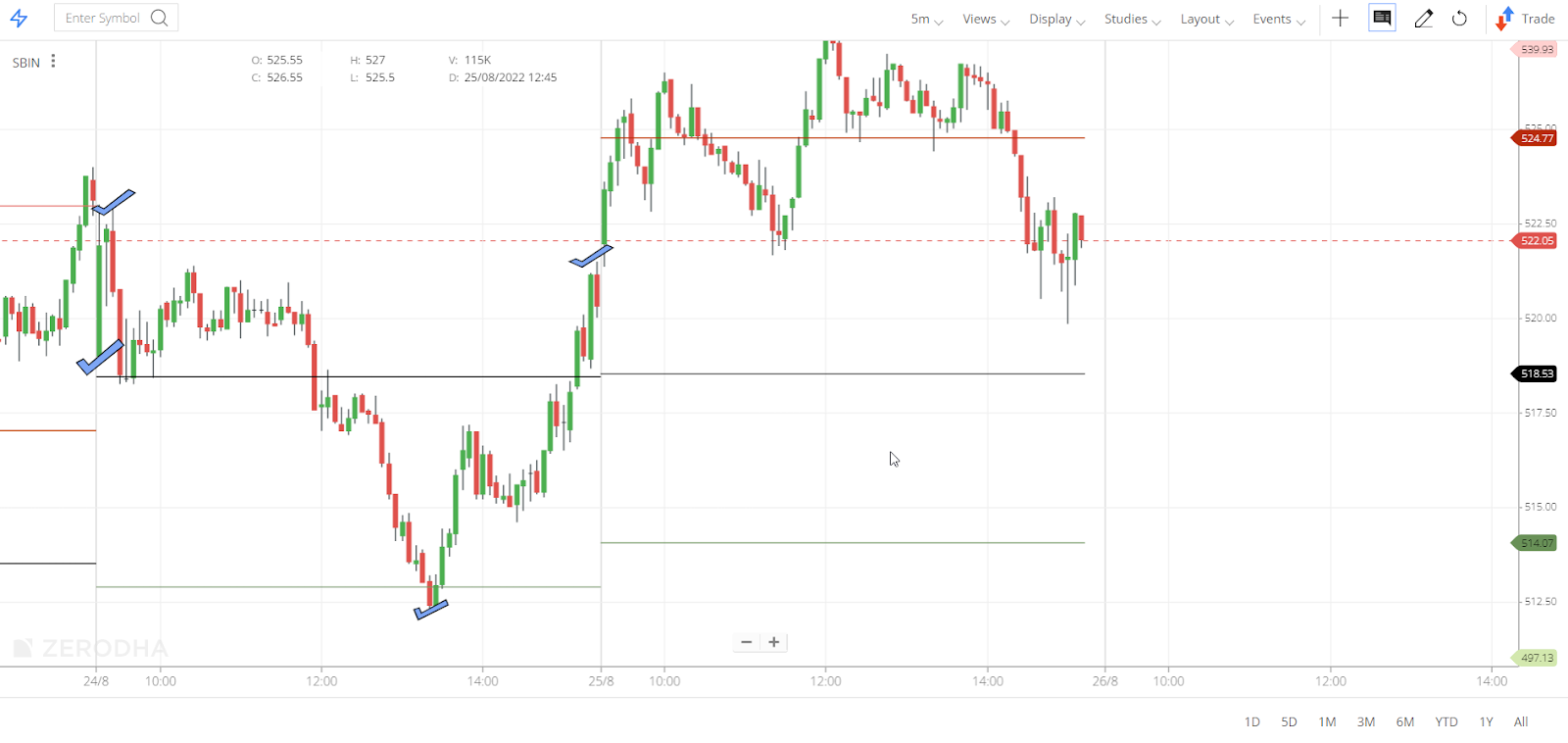##### Search for an answer or browse help topics to create a ticketView all categories
×

## How are the pivot points derived while using the Central Pivot Range (CPR)?

The Central Pivot Range (CPR) is an indicator to identify key price points to set up trades. The CPR consists of three components:

• Pivot
• Bottom Central Pivot (BC)
• Top Central Pivot (TC)

These are derived from the underlying high, low, and close price calculations:

• Pivot = (High + Low + Close) ÷ 3.
• Bottom CPR = (High + Low) ÷ 2.
• Top CPR = (Pivot - BC) + Pivot.

#### Example Scenario

• The chart of SBIN on 24/08/2022 (the previous day) has its Open (O) - 519, High (H) - 523, Low (L) - 512.3, and Close (C) - 519.3.
• On 25/08/2022, the pivot point data was 518.53. i.e. (H+L+C ÷ 3)
• Here if the pivot point data is ascertained from the OHLC data of 25/08/2022 it will not match with the pivot points as it is calculated using the previous day’s OHLC.The formula for calculating pivot points is mentioned below, with an example on Kite ChartIQ.

Assume XYZ’s stock has its High (H) - 129.7, Low (L) - 126.9 and Close (C) - 127.35, respectively. So the pivot points in Kite would be calculated as follows:

 KITE(ChartIQ) Pivot point = (H + L + C) / 3 127.98 First support level (S1) = (2 * P) – H 126.27 Second support level (S2) = P – (H – L) 125.18 Third support level (S3) = P – 2*(H-L) 122.38 First resistance level (R1) = (2 * P) – L 129.07 Second resistance level (R2) = P + (H – L) 130.78 Third resistance level (R3) = P + 2*(H – L) 133.58

Did you know? CPR helps the trader identify key price points and the associated trend around these price points. Today’s CPR values act as the reference for tomorrow’s trading.